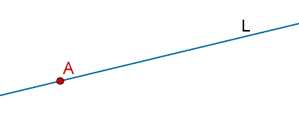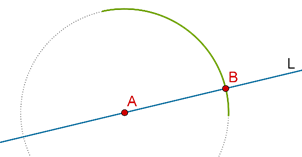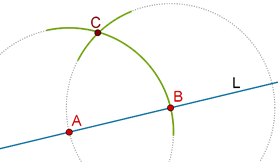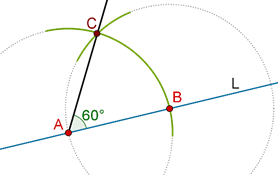# Constructing An Angle of 60 Degrees

Constructing An Angle of 60 Degrees
Go back to  'Angles'

Suppose that you have a line L and some point A on L:How will you construct a ray (or line) through A which is inclined at 600 to L?

Step 1: Taking A as center and any radius, draw an arc which intersects L at B:Step 2: Now, taking B as center and AB as radius, draw another arc which intersects the first arc at C:Step 3: Draw a ray (or line) through A and C. This will be inclined at 600 to L:Why does this construction work?

Proof: Note that AB = AC, since these are radii of the same circular arc. Also, BC = BA, since these too are radii of the same (second) circular arc. Thus,

AB = BC = AC

This means that $$\Delta ABC$$ is equilateral, and so, $$\angle BAC$$ = 600.

Note that you can construct an angle of 300 by bisecting an angle of 600, and you can further construct an angle of 150 by bisecting an angle of 300.

grade 9 | Questions Set 1